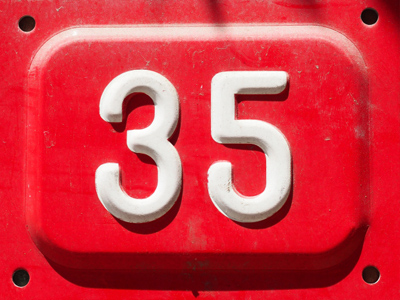5 x 7 = 35.

# 7 Times Table

This Math quiz is called '7 Times Table' and it has been written by teachers to help you if you are studying the subject at elementary school. Playing educational quizzes is an enjoyable way to learn if you are in the 3rd, 4th or 5th grade - aged 8 to 11.

It costs only \$12.50 per month to play this quiz and over 3,500 others that help you with your school work. You can subscribe on the page at Join Us

Multiplying numbers by 7 is what learning the 7 times table is about! You may wish to never see a times table again...but keep on practicing until you know every answer automatically. Learning your times tables will be beneficial in the long run - you'll find multiplying numbers much easier!

See how well you remember your 7 times table by playing this quiz.

1.
8 x 7 =
15
50
53
56
To work out 8 times a number, first times it by 10 and by 2. Then take away the x2 from the x10. So 7 x 10 = 70 and 7 x 2 = 14. 70 - 14 = 56
2.
6 x 7 =
28
34
42
43
3.
5 x 7 =
12
17
27
35
4.
4 x 7 =
26
28
32
33
5.
7 x 7 =
47
49
51
52
6.
2 x 7 =
9
12
14
17
14 days is two weeks, also known as a fortnight
7.
9 x 7 =
60
61
63
65
To times a number by 9 first times it by 10 and then take it away. So, 7 x 10 = 70 and 70 - 7 = 63
8.
3 x 7 =
18
20
21
23
21 is 7 + 7 + 7 or 3 + 3 + 3 + 3 + 3 + 3 + 3
9.
11 x 7 =
17
70
75
77
10 x 7 = 70 and 70 + 7 = 77
10.
12 x 7 =
77
84
88
92
10 x 7 = 70 and 2 x 7 = 14. 70 + 14 = 84
Author:  Amanda Swift

We use cookies to make your experience of our website better.

To comply with the new e-Privacy directive, we need to ask for your consent -